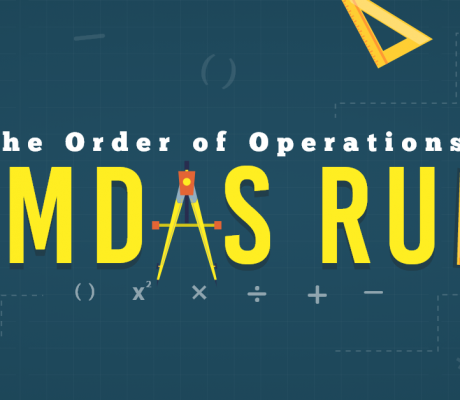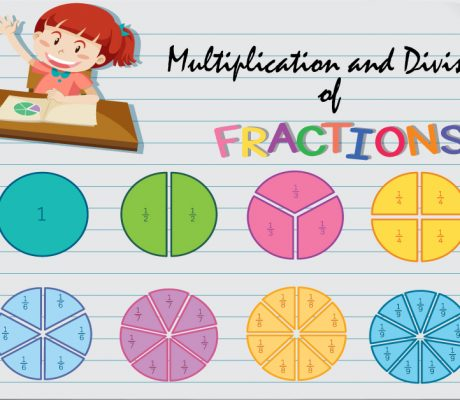# Educational Math Blogs### The Order of Operations: PEMDAS Rule

In Japan, the math problem went viral after a study found that only 60% of individuals in their 20’s were able to get the correct answer, lower than the 90% success rate in the 1980s. This study reflects the reality around the world that more and more students have given less importance to mastering the […]### Equivalent Fractions

Equivalent fractions are fractions that represent the same size even if the numbers look different. Let’s take a look at the pizzas below. The two fractions have different numerators and denominators, but they represent the same size of pizza slices. when simplified, is actually . It is important to remember that these two fractions still […]